# Investigating a Flood with EXCEL Feb 14 2014

• Slides: 30Investigating a Flood with EXCEL Feb 14, 2014 APEC Lesson Study Conference Hee-chan Lew & Chan-hee An Korea National University of Education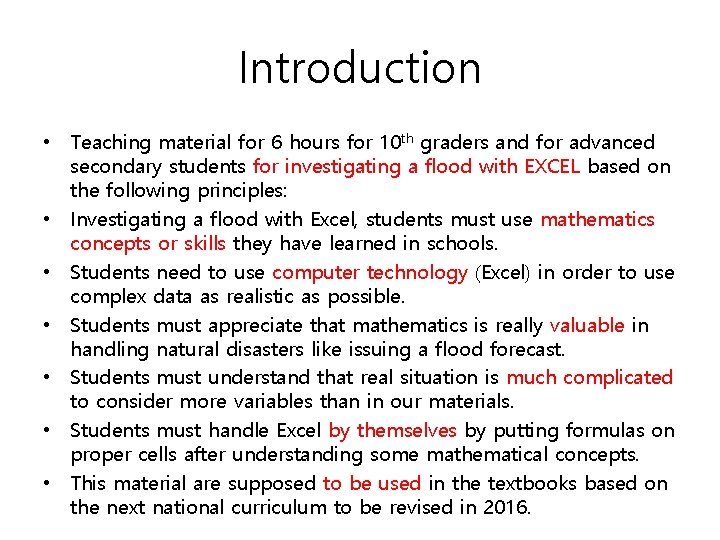Introduction • Teaching material for 6 hours for 10 th graders and for advanced secondary students for investigating a flood with EXCEL based on the following principles: • Investigating a flood with Excel, students must use mathematics concepts or skills they have learned in schools. • Students need to use computer technology (Excel) in order to use complex data as realistic as possible. • Students must appreciate that mathematics is really valuable in handling natural disasters like issuing a flood forecast. • Students must understand that real situation is much complicated to consider more variables than in our materials. • Students must handle Excel by themselves by putting formulas on proper cells after understanding some mathematical concepts. • This material are supposed to be used in the textbooks based on the next national curriculum to be revised in 2016.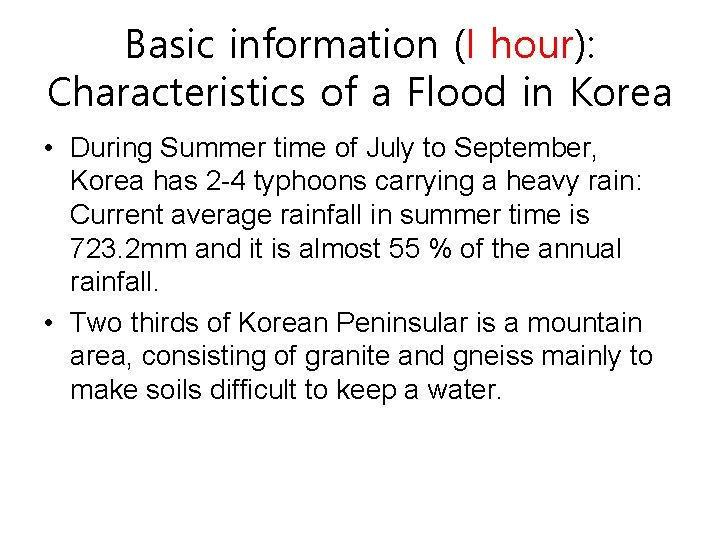Basic information (I hour): Characteristics of a Flood in Korea • During Summer time of July to September, Korea has 2 -4 typhoons carrying a heavy rain: Current average rainfall in summer time is 723. 2 mm and it is almost 55 % of the annual rainfall. • Two thirds of Korean Peninsular is a mountain area, consisting of granite and gneiss mainly to make soils difficult to keep a water.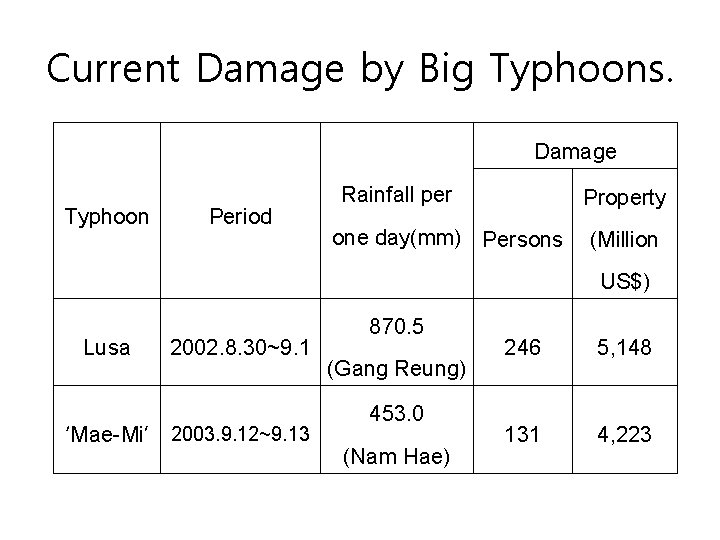Current Damage by Big Typhoons. Damage Typhoon Period Rainfall per Property one day(mm) Persons (Million US\$) Lusa 2002. 8. 30~9. 1 ‘Mae-Mi’ 2003. 9. 12~9. 13 870. 5 (Gang Reung) 453. 0 (Nam Hae) 246 5, 148 131 4, 223Process of a flood forecast • Korea Water Resources Corporation(KERC) • Functions of KERC: • It gets rainfall, an inflow and an outflow of main dams and rivers; measures water levels of main rivers; controls a storage of main dams or reservoirs in advance; issues a flood forecast • Here, students understand that some data for some key concepts are important to control a flood.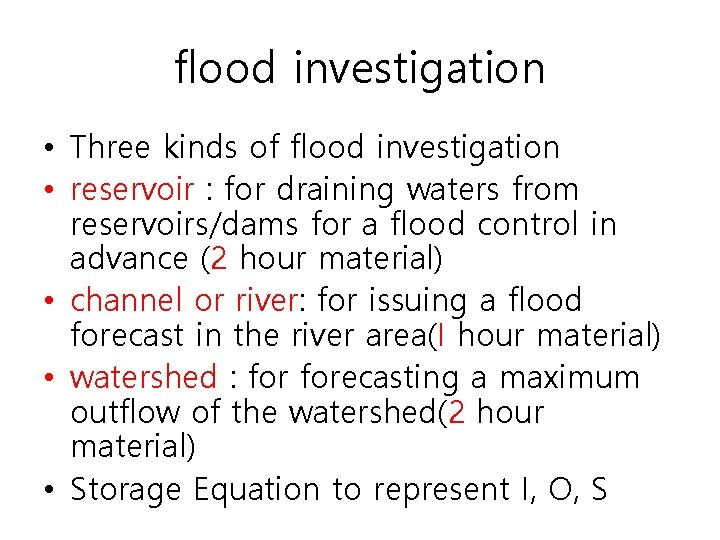flood investigation • Three kinds of flood investigation • reservoir : for draining waters from reservoirs/dams for a flood control in advance (2 hour material) • channel or river: for issuing a flood forecast in the river area(I hour material) • watershed : forecasting a maximum outflow of the watershed(2 hour material) • Storage Equation to represent I, O, S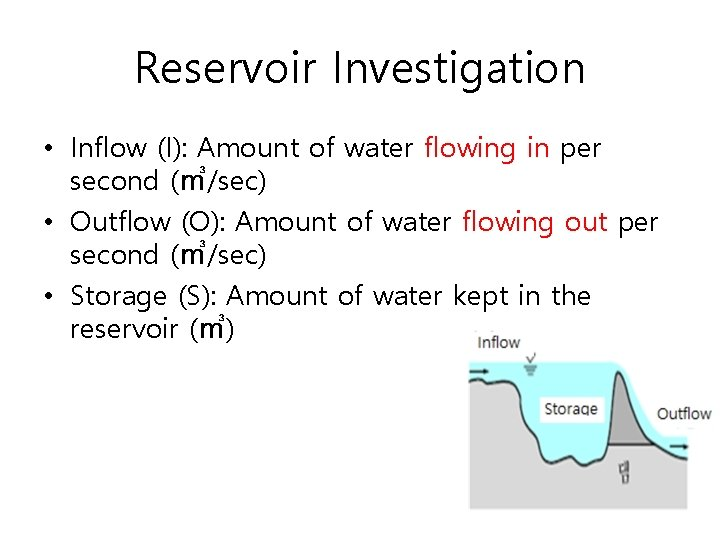Reservoir Investigation • Inflow (I): Amount of water flowing in per second (㎥/sec) • Outflow (O): Amount of water flowing out per second (㎥/sec) • Storage (S): Amount of water kept in the reservoir (㎥)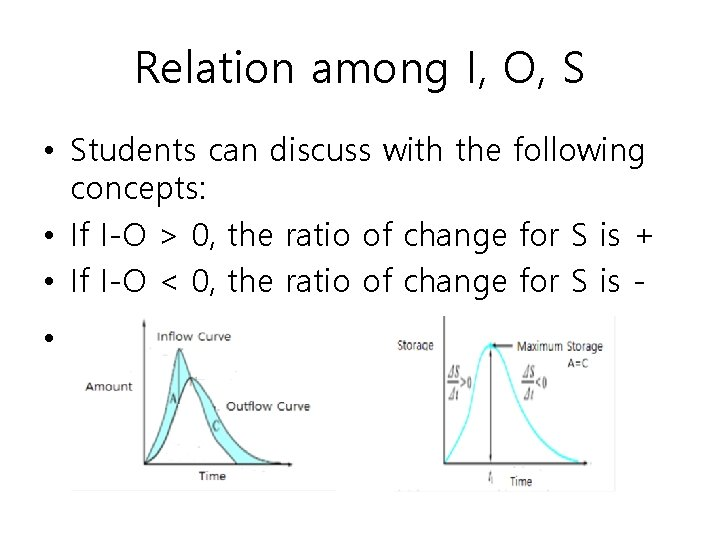Relation among I, O, S • Students can discuss with the following concepts: • If I-O > 0, the ratio of change for S is + • If I-O < 0, the ratio of change for S is •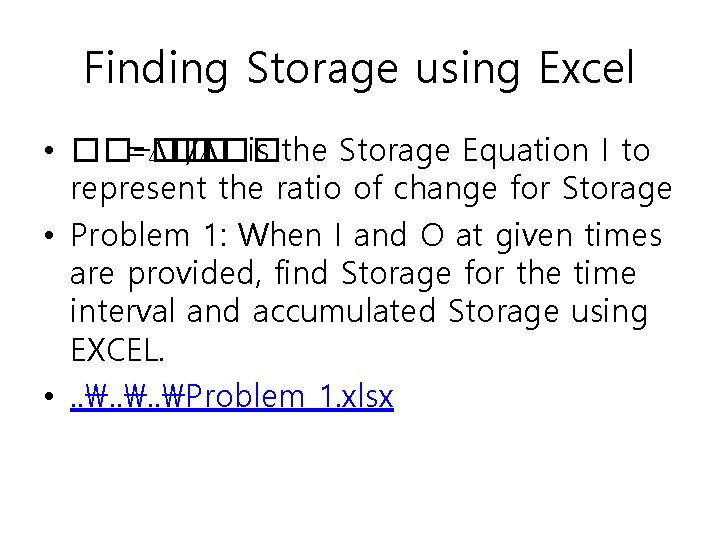Finding Storage using Excel • ��−�� =∆�� /∆�� is the Storage Equation I to represent the ratio of change for Storage • Problem 1: When I and O at given times are provided, find Storage for the time interval and accumulated Storage using EXCEL. • . . Problem 1. xlsx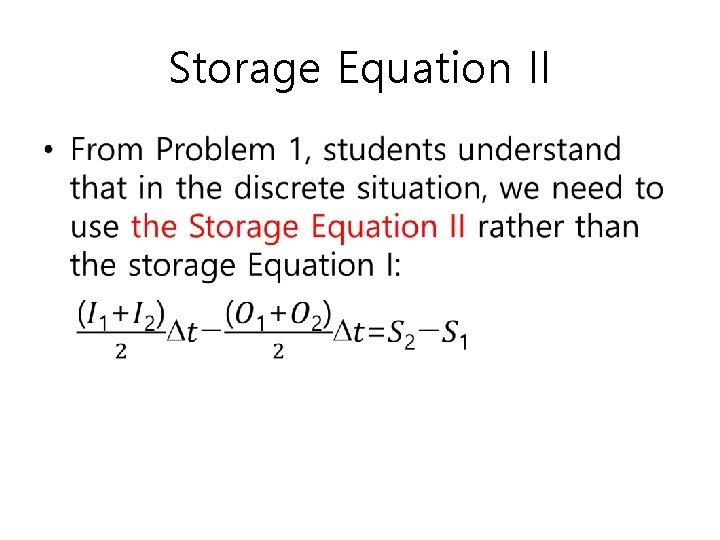Storage Equation II •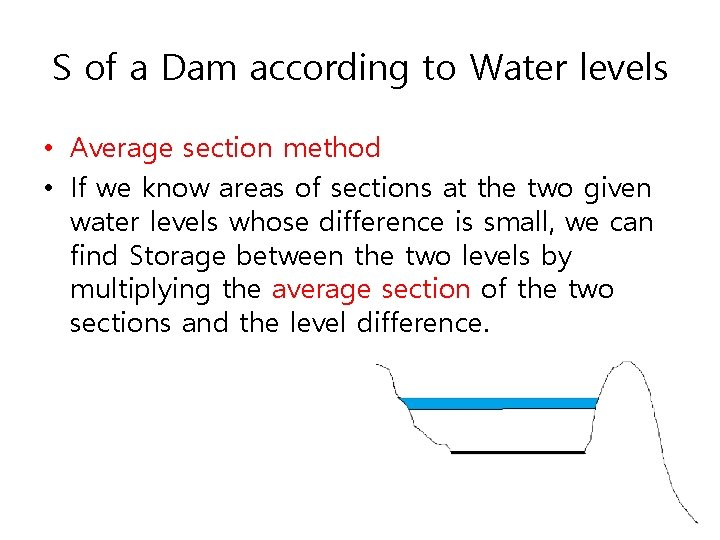S of a Dam according to Water levels • Average section method • If we know areas of sections at the two given water levels whose difference is small, we can find Storage between the two levels by multiplying the average section of the two sections and the level difference.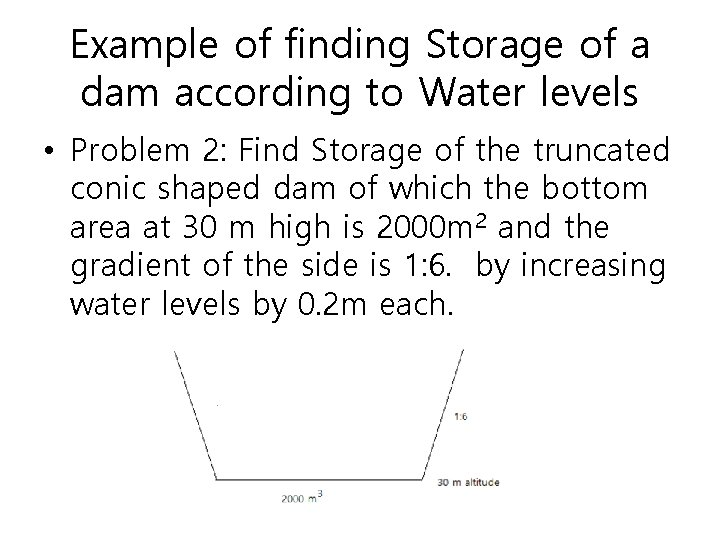Example of finding Storage of a dam according to Water levels • Problem 2: Find Storage of the truncated conic shaped dam of which the bottom area at 30 m high is 2000 m 2 and the gradient of the side is 1: 6. by increasing water levels by 0. 2 m each.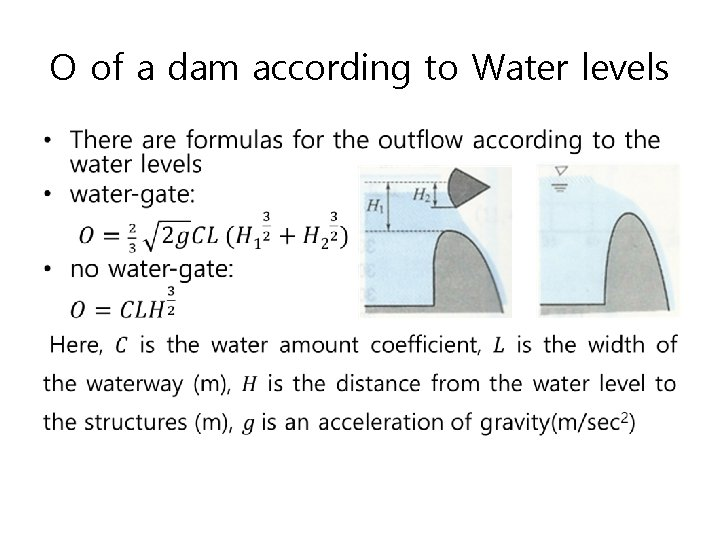O of a dam according to Water levels •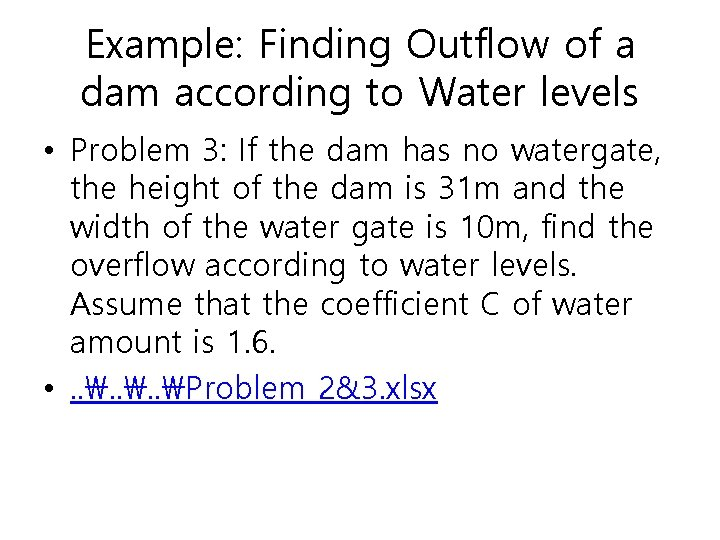Example: Finding Outflow of a dam according to Water levels • Problem 3: If the dam has no watergate, the height of the dam is 31 m and the width of the water gate is 10 m, find the overflow according to water levels. Assume that the coefficient C of water amount is 1. 6. • . . Problem 2&3. xlsx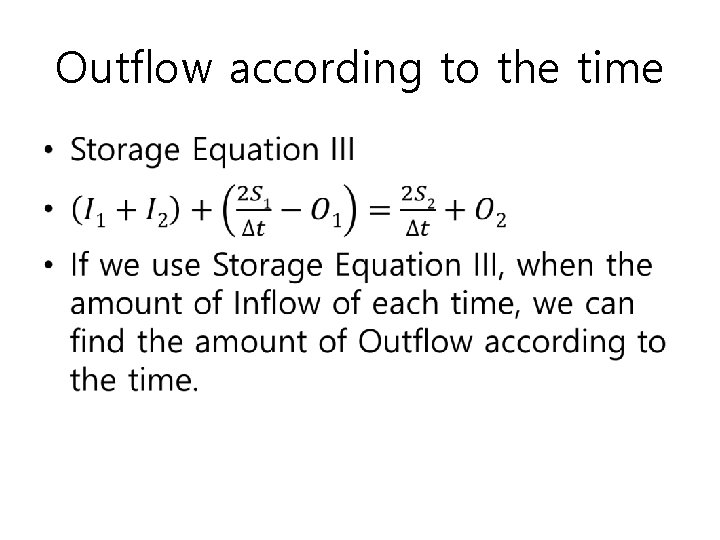Outflow according to the time •Example of Finding Outflow according to the time • Problem 4. There is a dam to keep water during flooding period. The bottom area of the dam is 4000㎡ and the dam has a vertical wall. When we know the data of I according to the given times, find O according to time. (We assume that the initial dam water amount is 0) • . . Problem 4. xlsx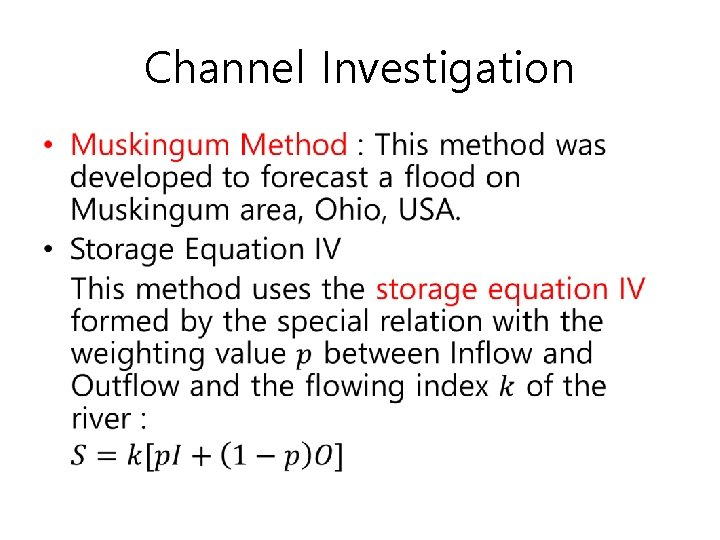Channel Investigation •Equation of Outflow •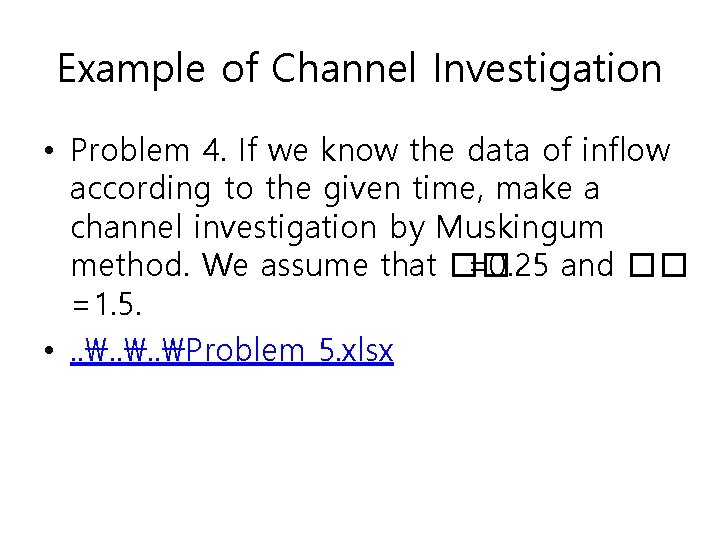Example of Channel Investigation • Problem 4. If we know the data of inflow according to the given time, make a channel investigation by Muskingum method. We assume that �� =0. 25 and �� =1. 5. • . . Problem 5. xlsx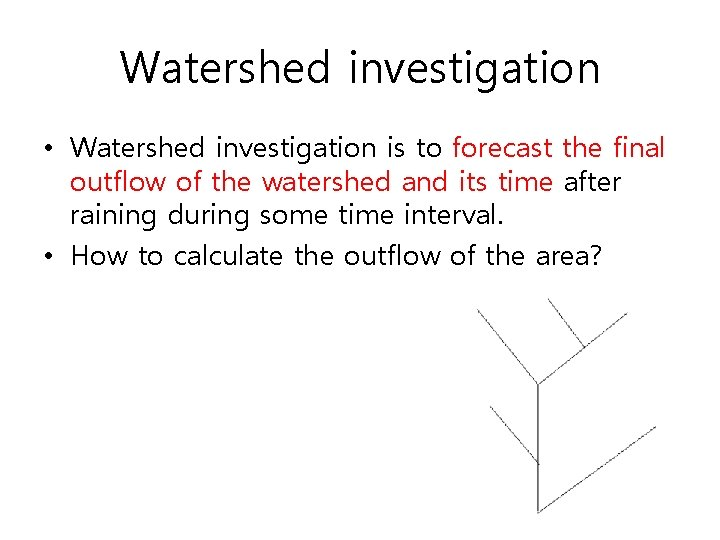Watershed investigation • Watershed investigation is to forecast the final outflow of the watershed and its time after raining during some time interval. • How to calculate the outflow of the area?Linear n dams • We have to assume that all amount of rainfall on the watershed flows through n hypothetical (non-exited) dams with linear arrangement in order to calculate outflow of the watershed for rainfall during some time interval.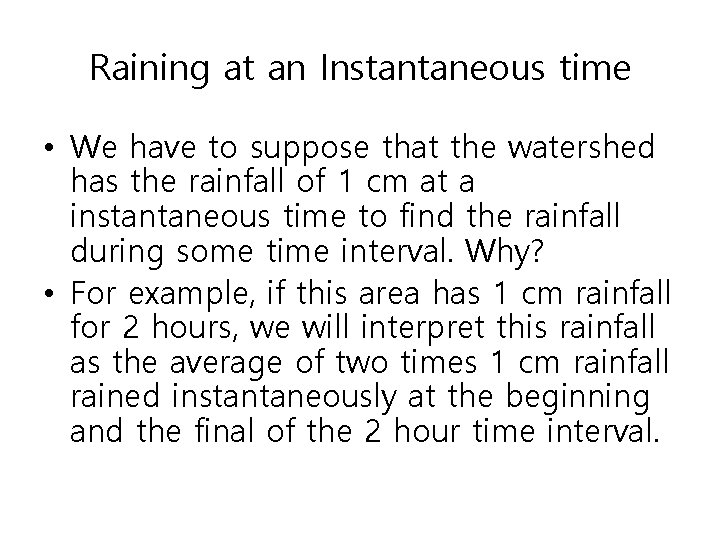Raining at an Instantaneous time • We have to suppose that the watershed has the rainfall of 1 cm at a instantaneous time to find the rainfall during some time interval. Why? • For example, if this area has 1 cm rainfall for 2 hours, we will interpret this rainfall as the average of two times 1 cm rainfall rained instantaneously at the beginning and the final of the 2 hour time interval.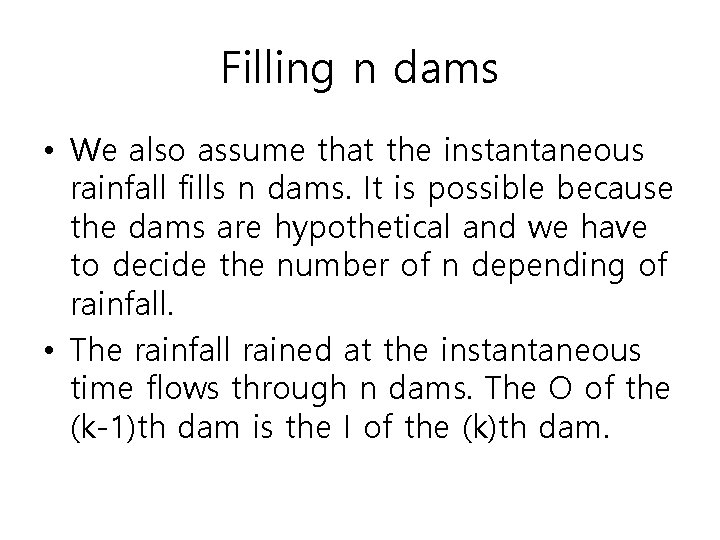Filling n dams • We also assume that the instantaneous rainfall fills n dams. It is possible because the dams are hypothetical and we have to decide the number of n depending of rainfall. • The rainfall rained at the instantaneous time flows through n dams. The O of the (k-1)th dam is the I of the (k)th dam.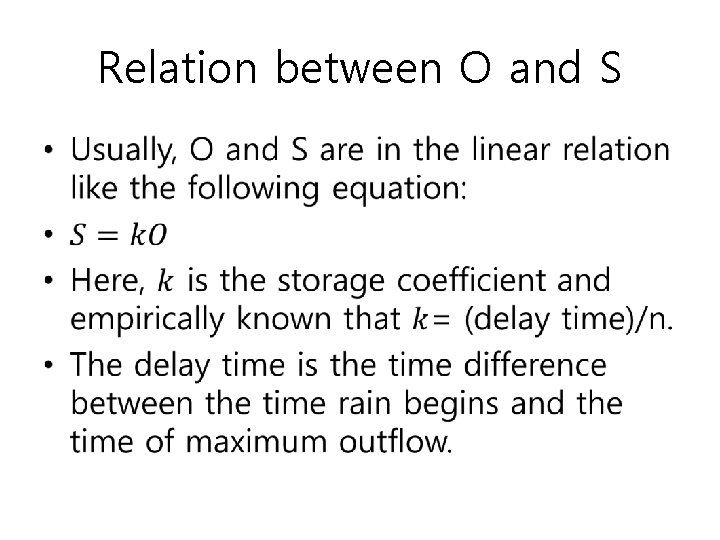Relation between O and S •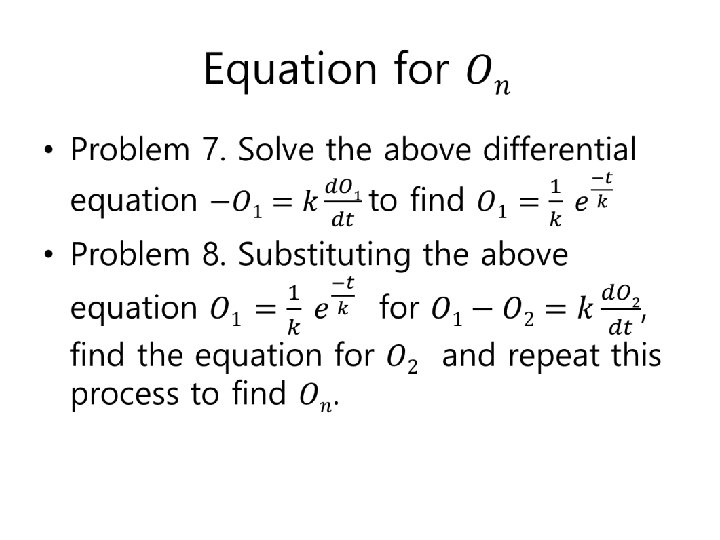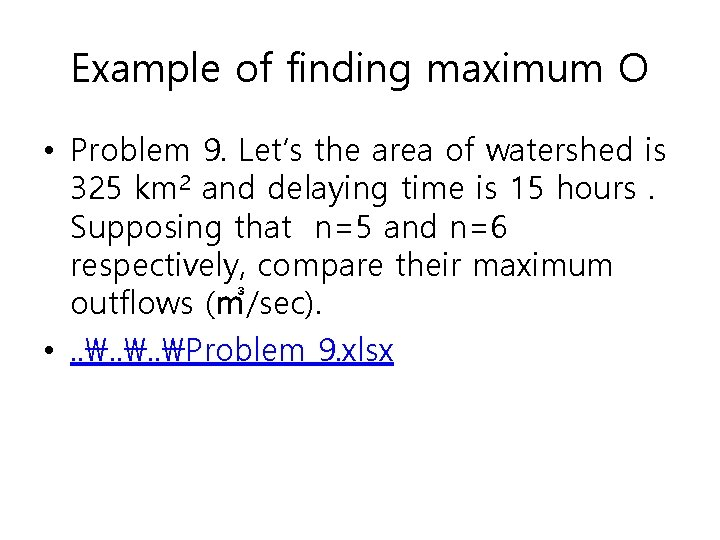Example of finding maximum O • Problem 9. Let’s the area of watershed is 325 km 2 and delaying time is 15 hours. Supposing that n=5 and n=6 respectively, compare their maximum outflows (㎥/sec). • . . Problem 9. xlsxmaximum outflow and its time Problem 10. Using the Excel data gotten in the case of n=5 in the problem 9, find the maximum outflow (㎥/sec) of the watershed and its time under the following condition. . . Problem 10. xlsx Rainfall Outflow (mm) coefficient 14: 00~1600 27. 3 0. 55 27. 3× 0. 55 ≒ 15. 0 16: 00~18: 00 56. 7 0. 60 56. 7× 0. 60 ≒ 34. 0 18: 00~20: 00 31. 4 0. 7 31. 4× 0. 7 ≒ 22. 0 date and time Aug 6 valid rainfall (mm)Epilog • To use EXCEL spontaneously, students must know enough mathematical concepts and skills to represent a flood situation in mathematical expression. • Excel is a very useful tool for modeling situations with mathematical concepts and skills. • This semester, I will use these materials for high school students and revise it. Then I will report the result at my society or at the next APEC meetings.